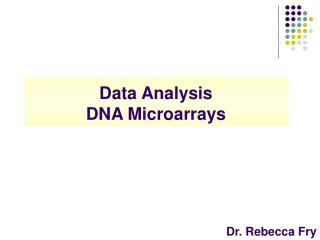DownloadDownload PresentationData Analysis DNA Microarrays

# Data Analysis DNA Microarrays

Télécharger la présentation## Data Analysis DNA Microarrays

- - - - - - - - - - - - - - - - - - - - - - - - - - - E N D - - - - - - - - - - - - - - - - - - - - - - - - - - -
##### Presentation Transcript

1. Data AnalysisDNA Microarrays Dr. Rebecca Fry

2. Raw Data File ID Cy3 Spot Mean Cy3 Background Cy5 mean Cy5 Background

3. Data Analysis • Step 1-Subtract Background Intensity from each spot

4. Generate Excel Formula

5. Paste into all cells

6. Data Analysis • Step 2-Caculate average cy3 and cy5 intensities for control spots to determine normalization factor

7. Sort File Based on Clone ID to locate controls

8. File is now alphabetized

9. Calculate average signals for cy3 and cy5

10. Calculate average signals for cy3 and cy5 cy3=32 cy5=9 cy3/cy5= 3.55

11. Normalization factor= cy3/cy5=# #=normalization factor Our data=3.55

12. Data Analysis • Step 3-Normalize data using cy5 adjustment factor

13. Paste into all cells

14. Data Analysis • Step 4-Filter Genes showing no expression in both channels • Filter Genes with ½ intensity (backgound subtracted, normalized) of the average control intensity in both channels

15. Generate Excel Formula

16. Paste into all cells

17. Filter genes that are not present in cy3 or cy5

18. Data Analysis • Step 5-Calculate Ratios of Gene expression change between final intensity value (background subtracted, normalized) Oligofectamine control (cy3) and knockdown (cy5) • Cy3/cy5=ratio=fold change

19. Why we filter!

20. Data Analysis • Step 6-Generate Summaries • Number of Genes with differential gene expression fold change above a cutoff (1.5) • Number of Genes expressed on array (need not be differential) • How do you explain number of genes expressed (biology/technology)? • How could you test this hypothesis?